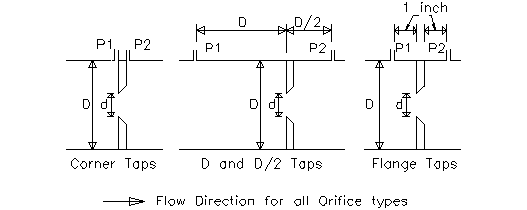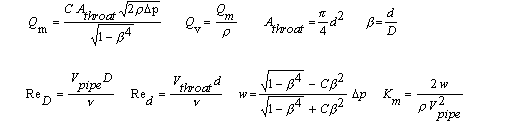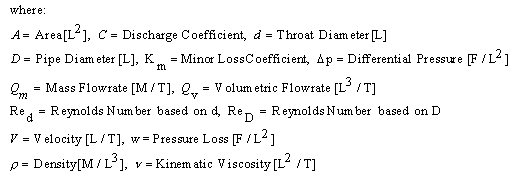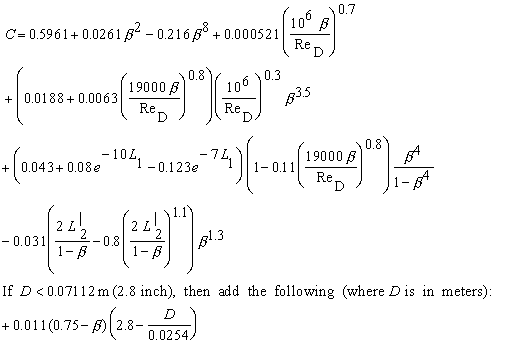Large Diameter Orifice Pipe Flow Rate Meter Calculation for Liquids

Pipe diameter > 5 cm. Compute pipe flow rate, orifice diameter, or differential pressure. Equations: ISO 5167

Register to enable "Calculate" button in pipe flow rate calculation.

 Volumetric Flow, Qv (m3/s): Mass Flow, Qm (kg/s): Solve for: Differential Pressure, Δp (Pa): Flowrate Differential Pressure. (Qv known) Differential Pressure. (Qm known) Throat Diameter. (Qv known) Throat Diameter. (Qm known) Throat Diameter, d (m): Pipe Diameter, D (m): Select Meter Type and Liquid: Ratio, d/D: Water at 70 F, 20 C Water at 40 F, 5 C Seawater at 60 F, 16 C SAE 30 oil at 60 F, 16 C User Defined Liquid Density (kg/m3): Corner Pressure Taps D and D/2 Pressure Taps Flange Pressure Taps Viscosity (m2/s): Discharge Coefficient, C: Select Units for each Variable: Reynolds No. based on d, Red: Qv in ft3/s or cfs Qv in ft3/min or cfm Qv in gal/min or gpm (U.S. gal) Qv in gal/hr or gph (U.S. gal) Qv in gal/day or gpd (U.S. gal) Qv in barrels/day Qv in m3/s Qv in m3/min Reynolds No. based on D, ReD: Qm in slug/s Qm in lb/s Qm in lb/hr Qm in kg/s Qm in kg/hr Pressure Loss, w (Pa): Pressure in psi Pressure in inch H2O (60F) Pressure in inch Mercury (60F) Pressure in millibars Pressure in N/m2 or Pa Pressure in mm Mercury (0C) Minor Loss Coefficient, Km: Diameters in inches Diameters in feet Diameters in cm Diameters in meters Throat Velocity, Vthroat (m/s): Density in slug/ft3 Density in lb/ft3 Density in kg/m3 Density in g/cm3 Density in N/m3 Pipe Velocity, Vpipe (m/s): Viscosity in ft2/s Viscosity in lb-s/ft2 Viscosity in m2/s Viscosity in N-s/m2 Viscosity in cP Viscosity in poise Viscosity in cSt Viscosity in stoke Throat Area, Athroat (m2): Velocity in ft/s; Area in ft2 Velocity in m/s; Area in m2 Pipe Area, Apipe (m2): ©2015 LMNO Engineering, Research, and Software, Ltd. www.LMNOeng.com

Units Orifice Pipe Flow Rate Meter calculator: cm=centimeter, ft=foot, g=gram, gal=U.S. gallon, hr=hour, kg=kilogram, km=kilometer, lb=pound, m=meter, min=minute, N=Newton, Pa=Pascal, psi=lb/inch2, s=second

Types of Pressure Taps for Orifices for Pipe Flow Rate Measurement:Topics on pipe flow rate page:  Introduction    Equations    Discharge Coefficients    Error Messages    References

Introduction to Pipe Flow Rate Measurement
Orifice pipe flow rate meters are used to determine a liquid or gas pipe flow rate by measuring the differential pressure (P1 - P2) across the orifice plate. Orifice meters are generally less expensive to install and manufacture than the other commonly used differential pressure pipe flow rate meters; however, nozzle and venturi pipe flow rate meters have the advantage of lower pressure drops. Equations for orifice meters have the advantage of no Reynolds Number upper limit for validity.

An orifice pipe flow rate meter is typically installed between flanges connecting two pipe sections (flanges are not shown in the above drawings). The three standard pressure tapping arrangements are shown in the drawings; the location of the pressure taps affects the discharge coefficient somewhat. Flange pressure taps penetrate the flange and are at a standard distance of 1 inch (2.54 cm) from either side of the orifice. For corner taps or D and D/2 taps, the pressure tap locations are as shown.

Orifices are typically less than 0.05D thick. For exact geometry and specifications for orifices, see ISO (1991) or ASME (1971). The ASME and ISO have been working on guidelines for orifices for pipe flow rate measurement since the early 1900s. The organizations have the most confidence in orifice accuracy when the Reynolds number exceeds 105, though Reynolds numbers as low as 4x103 are valid for certain d/D ratios as discussed below. The pipe flow rate calculation above is for liquids. Orifice gas pipe flow rate calculations (D<5 cm, D>5 cm) have an additional factor called expansibility.

Equations for Pipe Flow Rate Measurement
The pipe flow rate calculations on this page are for orifices carrying a liquid as described in ISO (1991) and ASME (1971). The ISO reference has a more up-to-date discussion of orifices than the ASME reference, so the ISO equations are used in our pipe flow rate calculations.k = Equivalent Roughness of the pipe material [L].

w is the static pressure loss occurring from a distance of approximately D upstream of the orifice to a distance of approximately 6D downstream of the orifice. It is not the same as differential pressure. Differential pressure is measured at the exact locations specified in ISO (1991) (shown in the above figures). Km is computed to allow you to design pipe systems with orifices and incorporate their head loss. Head loss is computed as h=KmV2/(2g) where V is the pipe velocity.

Discharge Coefficients for Pipe Flow Rate (ISO, 1998)
Equation and applicability:Corner Pressure Taps:  L1 = L'2 = 0
D and D/2 Pressure Taps:  L1 = 1  and  L'2 = 0.47
Flange Pressure Taps:  L1 = L'2 = 0.0254/D  where D is in meters

Applicability:
All types of pressure taps:  d ≥ 1.25 cm,  5 cm ≤ D ≤ 1 m,  0.1 ≤ d/D ≤ 0.75

Corner Pressure Taps or D and D/2 Pressure Taps:
ReD ≥ 4000  for  0.1 ≤ d/D ≤ 0.5   and  ReD ≥ 16,000(d/D )2  for  d/D>0.5

Flange Pressure Taps:  ReD ≥ 4000  and  ReD ≥ 170,000 D (d/D )2  where D is in meters

In addition, ISO recommends that in general  k/D ≤ 3.8x10-4   for Corner Taps and  k/D ≤ 10-3 for Flange or D and D/2 pressure taps.  k is the pipe roughness.

Error Messages given by pipe flow rate calculation
"All inputs must be positive". This is an initial check of user input to the pipe flow rate calculator.
"d, D, d/D, or ReD out of range". The equation for discharge coefficient, C, is only valid for certain ranges of d, D, d/D, and ReD as shown in the Discharge Coefficients section above. This message could be generated during the pipe flow rate program's initial check for valid input. If the input is valid, the message will be generated during computations if the program determines that a calculated value will be out of range of the validity of the equations. d is orifice diameter, D is pipe diameter, and ReD is the Reynolds number based on D.
"ReD is too small". During computations, it has been determined that ReD will not be large enough to satisfy the validity equations.
Try the simpler orifice pipe flow rate calculation on our Bernoulli page if your parameters are out of range. It is not as accurate, but won't give "parameter out of range" error messages.

References for orifice pipe flow rate meters
American Society of Mechanical Engineers (ASME). 1971. Fluid meters: Their theory and application. Edited by H. S. Bean. 6ed. Report of ASME Research Committee on Fluid Meters.

International Organization of Standards (ISO 5167-1). 1991. Measurement of fluid flow by means of pressure differential devices, Part 1: Orifice plates, nozzles, and Venturi tubes inserted in circular cross-section conduits running full. Reference number: ISO 5167-1:1991(E).

International Organization of Standards (ISO 5167-1) Amendment 1. 1998. Measurement of fluid flow by means of pressure differential devices, Part 1: Orifice plates, nozzles, and Venturi tubes inserted in circular cross-section conduits running full. Reference number: ISO 5167-1:1991/Amd.1:1998(E).

LMNO Engineering, Research, and Software, Ltd.
7860 Angel Ridge Rd.   Athens, Ohio  45701  USA   Phone: (740) 592-1890
LMNO@LMNOeng.com    http://www.LMNOeng.com

To:

Other Flow Meter Calculations using standard methodologies:

Orifice for liquids (D<5cm)

Orifice for gases (D<5 cm)

Orifice for gases (D>5cm)

Nozzle for liquids

Venturi for liquids

Simpler orifice calculation (not as accurate but won't give "parameter out of range" messages):

Bernoulli page

Unit Conversions

Register